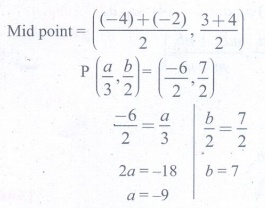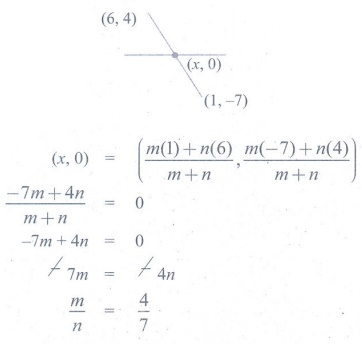Home | | Maths 9th std | Exercise 5.6: Multiple Choice Questions

# Exercise 5.6: Multiple Choice Questions

Maths : Coordinate Geometry: Book Back, Exercise, Example Numerical Question with Answers, Solution: Exercise 5.6: Multiple Choice Questions, One Mark Choose the correct Answers

Coordinate Geometry

Exercise 5.6

Multiple Choice Questions

1. If the y-coordinate of a point is zero, then the point always lies ______

(3) on x-axis

(4) on y-axis

Solution:

Points in x - axis has y - coordinate zero

2. The points (–5, 2) and (2, –5) lie in the ________

(2) II and III quadrant respectively

(3) II and IV quadrant respectively

(4) IV and II quadrant respectively

Solution:

3. On plotting the points O(0,0), A(3, – 4), B(3, 4) and C(0, 4) and joining OA, AB, BC and CO, which of the following figure is obtained?

(1) Square

(2) Rectangle

(3) Trapezium

(4) Rhombus

Solution:

In trapezium one pair of opposite side is parallel.

4. If P( –1,1), Q( 3,–4), R( 1, –1), S(–2, –3) and T( –4, 4) are plotted on a graph paper, then the points in the fourth quadrant are __________

(1) P and T

(2) Q and R

(3) only S

(4) P and Q

Solution:

Points in IVth quadrant are (+,)

5. The point whose ordinate is 4 and which lies on the y-axis is _______________

(1)( 4, 0 )

(2) (0, 4)

(3) (1, 4)

(4) (4, 2)

Solution:

Points in y-axis have abscissa a zero

6. The distance between the two points ( 2, 3 ) and ( 1, 4 ) is ______

(1) 2

(2) √56

(3)  √10

(4) √2

Solution:

Distance = √[(x2x1)2+(y2y1)2]

7. If the points A (2,0), B (-6,0), C (3, a–3) lie on the x-axis then the value of a is _____

(1) 0

(2) 2

(3) 3

(4) –6

Solution:

Points in y-axis have ordinate zero.

a3 = 0

a = 3

8. If ( x+2, 4) = (5, y–2), then the coordinates (x,y) are _____

(1) (7, 12)

(2) (6, 3)

(3) (3, 6)

(4) (2, 1)

Solution:

x + 2 = 5 x = 3; y 2 = 4 y = 6

9. If Q1, Q2, Q3, Q4 are the quadrants in a Cartesian plane then Q2 ∩ Q3 is ___________

(1) Q1∩ Q2

(2) Q2∩Q3

(3) Null set

(4) Negative x-axis.

Solution:

Quadrants do not contain the axis.

10. The distance between the point ( 5, –1 ) and the origin is _________

(1) √24

(2) √37

(3) √26

(4) √17

Solution:

Distance = [(5-0)2 + (1 0)2] = √[52+l2] = 26

11. The coordinates of the point C dividing the line segment joining the points P(2,4) and Q(5,7) internally in the ratio 2:1 is

(1) (7/2, 11/2)

(2) (3,5)

(3) (4,4)

(4) (4,6)

Solution:12. If P (a/3,b/2) is the mid-point of the line segment joining A(−4,3) and B(−2,4) then (a,b) is

(1) (−9,7)

(2) (−3, 7/2)

(3) (9, −7)

(4) ( 3, −7/2 )

Solution:13. In what ratio does the point Q(1,6) divide the line segment joining the points P(2,7) and R(−2,3)

(1) 1:2

(2) 2:1

(3) 1:3

(4) 3:1

Solution:14. If the coordinates of one end of a diameter of a circle is (3,4) and the coordinates of its centre is (−3,2), then the coordinate of the other end of the diameter is

(1) (0,−3)

(2) (0,9)

(3) (3,0)

(4) (−9,0)

Solution:15. The ratio in which the x-axis divides the line segment joining the points A(a1 ,b1 ) and B (a2 ,b2 ) is

(1) b1 : b2

(2) b1 : b2

(3) a1 : a2

(4) a1 : a2

Solution:16. The ratio in which the x-axis divides the line segment joining the points (6,4) and (1, −7) is

(1) 2:3

(2) 3:4

(3) 4:7

(4) 4:3

Solution:17. If the coordinates of the mid-points of the sides AB, BC and CA of a triangle are (3,4), (1,1) and (2,−3) respectively, then the vertices A and B of the triangle are

(1) (3,2), (2,4)

(2) (4,0), (2,8)

(3) (3,4), (2,0)

(4) (4,3), (2,4)

Solution:18. The mid-point of the line joining (−a,2b) and (−3a,−4b) is

(1) (2a,3b)

(2) (−2a, −b)

(3) (2a,b)

(4) (−2a, −3b)

Solution:19. In what ratio does the y-axis divides the line joining the points (−5,1) and (2,3) internally

(1) 1:3

(2) 2:5

(3) 3:1

(4) 5:2

Solution:20. If (1,−2), (3,6), (x,10) and (3,2) are the vertices of the parallelogram taken in order, then the value of x is

(1) 6

(2) 5

(3) 4

(4) 3

Solution:

In parallelogram diagonals bisect each other.

Mid point of AC = Mid point of BD6 (2) / 31 = 102 / x−3

8/2 = 8/x−3

x−3 = 2

x = 5